Worksheet on Construction on Quadrilateral is the first source for every student who wishes to learn complete concepts of Construction of Quadrilateral. You can see different problems along with answers, and explanations where you can find different processes to solve one problem. Practice all the problems and improve your preparation level easily. Quadrilateral Worksheet is included on our website with practice questions and solved problems.

1. Construct a quadrilateral PQRS in which PQ = 4.4 cm, QR = 6.2 cm, RS = 5.4 cm, SP = 5.2 cm And PR = 8.2 cm.

Solution:

Steps of Construction:
Firstly, draw a rough figure of the quadrilateral with the given dimensions.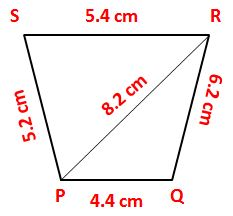1. Draw a line segment of length 4.4 cm and mark the ends as P and Q.
2. Take the point P as a center and draw an arc by taking the radius 8.2 cm.
3. Next, take point Q as a center and draw an arc by taking the radius 6.2 cm. Mark the point as R where the two arcs cross each other. Join the points Q and R as well as P and R.
4. By taking the point P as a center, draw an arc with a radius of 5.2 cm.
5. By taking the point R as a center, draw an arc with a radius of 5.4 cm.
6. Mark the point as S where the two arcs cross each other. Join the points R and S as well as P and S.

The final result is the required quadrilateral.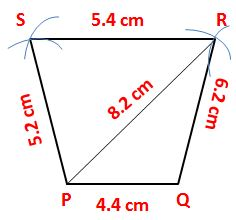2. Construct a quadrilateral ABCD in which AB = 5.4 cm, BC = 4.6 cm, CD = 4.3 cm, DA = 3.5 cm and diagonal AC = 5.6 cm

Solution:

Steps of Construction:
Given that a quadrilateral ABCD in which AB = 5.4 cm, BC = 4.6 cm, CD = 4.3 cm, DA = 3.5 cm and diagonal AC = 5.6 cm
1. Draw a line segment of length 5.6 cm and mark the ends as A and C.
2. Take point A as a center and draw an arc by taking the radius 5.4 cm above the diagonal.
3. Next, take point C as a center and draw an arc by taking the radius 4.6 cm above the diagonal. Mark the point as B where the two arcs cross each other. Join the points A and B as well as B and C.
4. By taking point A as a center, draw an arc with a radius of 3.5 cm below the diagonal.
5. By taking the point C as a center, draw an arc with a radius of 4.3 cm below the diagonal.
6. Mark the point as D where the two arcs cross each other. Join the points A and D as well as C and D.

The final result is the required quadrilateral.3. Construct a quadrilateral PQRS in which PQ = 3.5 cm, QR = 3.8 cm, RS = SP = 4.5 cm and diagonal QR = 5.6 cm.

Solution:

Steps of Construction:
Given that a quadrilateral PQRS in which PQ = 3.5 cm, QR = 3.8 cm, RS = SP = 4.5 cm and diagonal QR = 5.6 cm.
1. Draw a line segment of length 5.6 cm and mark the ends as S and R.
2. Take point S as a center and draw an arc by taking the radius 5.6 cm.
3. Next, take point R as a center and draw an arc by taking the radius 3.8 cm. Mark the point as Q where the two arcs cross each other. Join the points S and Q as well as R and Q.
4. By taking point S as a center, draw an arc with a radius of 4.5 cm.
5. By taking the point Q as a center, draw an arc with a radius of 3.5 cm.
6. Mark the point as P where the two arcs cross each other. Join the points Q and P as well as S and P.

The final result is the required quadrilateral.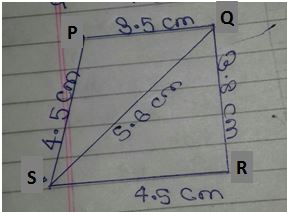4. Construct a quadrilateral PQRS in which PQ = 3.6 cm, QR = 3.3 cm, PS = 2.7 cm, diagonal PR = 4.6 cm and diagonal QS = 4 cm.

Solution:

Steps of Construction:
Given that a quadrilateral PQRS in which PQ = 3.5 cm, QR = 3.8 cm, RS = SP = 4.5 cm and diagonal QR = 5.6 cm.
1. Draw a line segment of length 3.6 cm and mark the ends as P and Q.
2. Take point P as a center and draw an arc by taking the radius 4.6 cm.
3. Next, take point Q as a center and draw an arc by taking the radius 3.3 cm. Mark the point as R where the two arcs cross each other. Join the points P and R as well as R and Q.
4. By taking point P as a center, draw an arc with a radius of 2.7 cm.
5. By taking the point Q as a center, draw an arc with a radius of 4 cm.
6. Mark the point as S where the two arcs cross each other. Join the points Q and S as well as S and P.

The final result is the required quadrilateral.5. Construct a quadrilateral ABCD in which AC = AD = 6 cm, BC = 7.5 cm , BD = 10 cm and CD = 5 cm. Measure the remaining side.

Solution:

Steps of Construction:
Given that a quadrilateral ABCD in which AC = AD = 6 cm, BC = 7.5 cm , BD = 10 cm and CD = 5 cm. Measure the remaining side.
1. Draw a line segment of length 6 cm and mark the ends as A and D.
2. Take point A as a center and draw an arc by taking the radius 6 cm.
3. Next, take point D as a center and draw an arc by taking the radius 5 cm. Mark the point as C where the two arcs cross each other. Join the points D and C.
4. By taking point D as a center, draw an arc with a radius of 10 cm.
5. By taking the point C as a center, draw an arc with a radius of 7.5 cm.
6. Mark the point as B where the two arcs cross each other. Join the points B and C as well as B and D.

The final result is the required quadrilateral.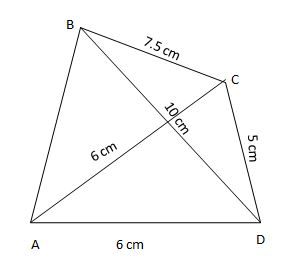6. Construct a quadrilateral PQRS in which PQ = 3.4 cm, RS = 3 cm, SP = 5.7 cm, PR = 8 cm and QS = 4 cm.

Solution:

Steps of Construction:
Given that a quadrilateral PQRS in which PQ = 3.4 cm, RS = 3 cm, SP = 5.7 cm, PR = 8 cm and QS = 4 cm.
1. Draw a line segment of length 3.4 cm and mark the ends as P and Q.
2. Take the point Q as a center and draw an arc by taking the radius 4 cm.
3. Next, take point P as a center and draw an arc by taking the radius 5.7 cm. Mark the point as S where the two arcs cross each other. Join the points Q and S as well as P and S.
4. By taking the point P as a center, draw an arc with a radius of 8 cm.
5. By taking the point S as a center, draw an arc with a radius of 3 cm.
6. Mark the point as R where the two arcs cross each other. Join the points R and S as well as P and R.

The final result is the required quadrilateral.7. Construct a quadrilateral PQRS in which PQ = QR = 3.5 cm, PS = RS = 5.2 cm and ∠PQR = 120°.

Solution:

Steps of Construction:
Given that PQ = QR = 3.5 cm, PS = RS = 5.2 cm and ∠PQR = 120°.
1. Draw a line segment of length 3.5 cm and mark the ends as P and Q.
2. Take point Q as a center and make a point by taking 120º using a protector.
3. Next, take point Q as a center and draw an arc with a radius of 3.5 cm. Mark the point as R where the two points are meet at a point. Join the points Q and R.
4. By taking the point P as a center, draw an arc with a radius of 5.2 cm.
5. By taking the point R as a center, draw an arc with a radius of 5.2 cm.
6. Mark the point as S where the two arcs cross each other. Join the points P and S, R and S.

The final result is the required quadrilateral.8. Construct a quadrilateral PQRS in which PQ = 2.9 cm, QR = 3.2 cm, RS = 2.7 cm, SP = 3.4 cm and ∠P = 70°.

Solution:

Steps of Construction:
Given that a quadrilateral PQRS in which PQ = 2.9 cm, QR = 3.2 cm, RS = 2.7 cm, SP = 3.4 cm and ∠P = 70°.
1. Draw a line segment of length 2.9 cm and mark the ends as P and Q.
2. Take point P as a center and make a point by taking 70º using a protector.
3. Next, take point P as a center and draw an arc with a radius of 3.4 cm. Mark the point as S where the two points are meet at a point. Join the points P and S.
4. By taking the point S as a center, draw an arc with a radius of 2.7 cm.
5. By taking the point Q as a center, draw an arc with a radius of 3.2 cm.
6. Mark the point as R where the two arcs cross each other. Join the points Q and S, R and S.

The final result is the required quadrilateral.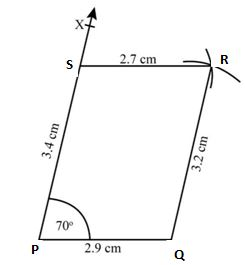9. Construct a quadrilateral PQRS in which PQ = 3.5 cm, QR = 5 cm, RS = 4.6 cm, ∠Q = 125° and ∠R = 60°.

Solution:

Steps of Construction:
Given that a quadrilateral PQRS in which PQ = 3.5 cm, QR = 5 cm, RS = 4.6 cm, ∠Q = 125° and ∠R = 60°.
1. Draw a line segment of length 5 cm and mark the ends as Q and R.
2. Take point Q as a center and make a point by taking 125º using a protector.
3. Next, take point R as a center and make a point by taking 60º using a protector.
4. By taking the point Q as a center, draw an arc with a radius of 3.5 cm.
5. Mark the point as P where the two points are meet at a point. Join the points Q and P.
6. By taking the point R as a center, draw an arc with a radius of 4.6 cm.
7. Mark the point as S where the point and arc cross each other. Join the points P and S.

The final result is the required quadrilateral.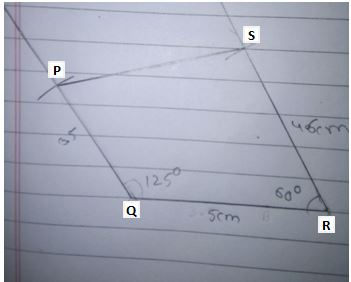10. Construct a quadrilateral ABCD in which AB = 6 cm, BC = 5.6 cm, CD = 2.7 cm, ∠B = 45° and ∠C = 90°.

Solution:

Steps of Construction:
Given that a quadrilateral ABCD in which AB = 6 cm, BC = 5.6 cm, CD = 2.7 cm, ∠B = 45° and ∠C = 90°.
1. Draw a line segment of length 6 cm and mark the ends as A and B.
2. Take point B as a center and make a point by taking 45º using a protector.
3. By taking the point B as a center, draw an arc with a radius of 5.6 cm.
5. Mark the point as C where the point and arc are meet at a point. Join the points B and C.
6. By taking the point C as a center, draw an arc with a radius of 2.7 cm.
7. Take point C as a center and make a point by taking 90º using a protector.
7. Mark the point as D where the point and arc cross each other. Join the points D and A, D and C.

The final result is the required quadrilateral.11. Construct a quadrilateral PQRS in which PQ = 5.6 cm, QR = 4 cm, ∠P = 50°, ∠Q = 105° and ∠S = 80°.

Solution:

Steps of Construction:
Given that a quadrilateral PQRS in which PQ = 5.6 cm, QR = 4 cm, ∠P = 50°, ∠Q = 105° and ∠S = 80°.
We know that ∠P + ∠Q + ∠R + ∠S = 360°
So, 50° + 105° + ∠R + 80° = 360°
∠R = 125°
1. Draw a line segment of length 5.6 cm and mark the ends as P and Q.
2. Take point P as a center and make a point by taking 50º using a protector. Also, take point Q as a center and make a point by taking 150º using a protector.
3. By taking the point Q as a center, draw an arc with a radius of 4 cm.
5. Mark the point as R where the point and arc are meet at a point. Join the points Q and R.
6. By taking the point R as a center, make a point by taking 125º using a protector.
7. Mark the point as S where the point and arc cross each other. Join the points S and P, S and R.

The final result is the required quadrilateral.12. Construct a quadrilateral ABCD in which AB = 5 cm, BC = 6.5 cm, ∠A = ∠C = 100° and ∠D = 75°.

Solution:

Steps of Construction:
Given that a quadrilateral ABCD in which AB = 5 cm, BC = 6.5 cm, ∠A = ∠C = 100° and ∠D = 75°.
We know that ∠A + ∠B + ∠C + ∠D = 360°
So, 100° + ∠B + 100° + 75° = 360°
∠B = 125°
1. Draw a line segment of length 5 cm and mark the ends as A and B.
2. Take point A as a center and make a point by taking 100º using a protector. Also, take point B as a center and make a point by taking 125º using a protector.
3. By taking the point B as a center, draw an arc with a radius of 6.5 cm.
5. Mark the point as C where the point and arc are meet at a point. Join the points B and C.
6. By taking the point C as a center, make a point by taking 100º using a protector.
7. Mark the point as D where the point and arc cross each other. Join the points D and A, D and C.

The final result is the required quadrilateral.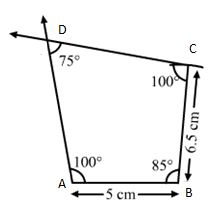13. Construct a quadrilateral PQRS in which PQ = 4 cm, PR = 5 cm, PS = 5.5 cm and ∠PQR = ∠PRS = 90°.

Solution:

Steps of Construction:
Given that a quadrilateral PQRS in which PQ = 4 cm, PR = 5 cm, PS = 5.5 cm and ∠PQR = ∠PRS = 90°.
1. Draw a line segment of length 4 cm and mark the ends as P and Q.
2. Take point Q as a center and make a point by taking 90º using a protector. Also, take point P as a center and make a point by taking 90º using a protector.
3. By taking the point P as a center, draw an arc with a radius of 5 cm.
5. Mark the point as R where the point and arc are meet at a point. Join the points Q and R.
6. By taking the point P as a center, draw an arc with a radius of 5.5 cm.
7. Mark the point as S where the point and arc cross each other. Join the points S and P, S and R.

The final result is the required quadrilateral.